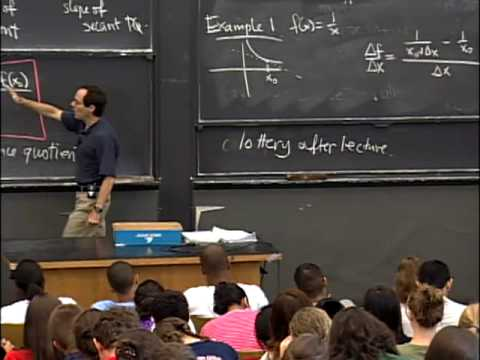MIT OpenCourseWare

# Single Variable Calculus

This is an introductory calculus course covering differentiation and integration of functions of one variable, with applications: differentiation, application of differentiation, definite integral and its applications, techniques of integration, and a brief discussion of infinite series. (from ocw.mit.edu )

Instructor

Mathematics
2009

#### Lecture 01 - Derivatives, Slope, Velocity, Rate of Change